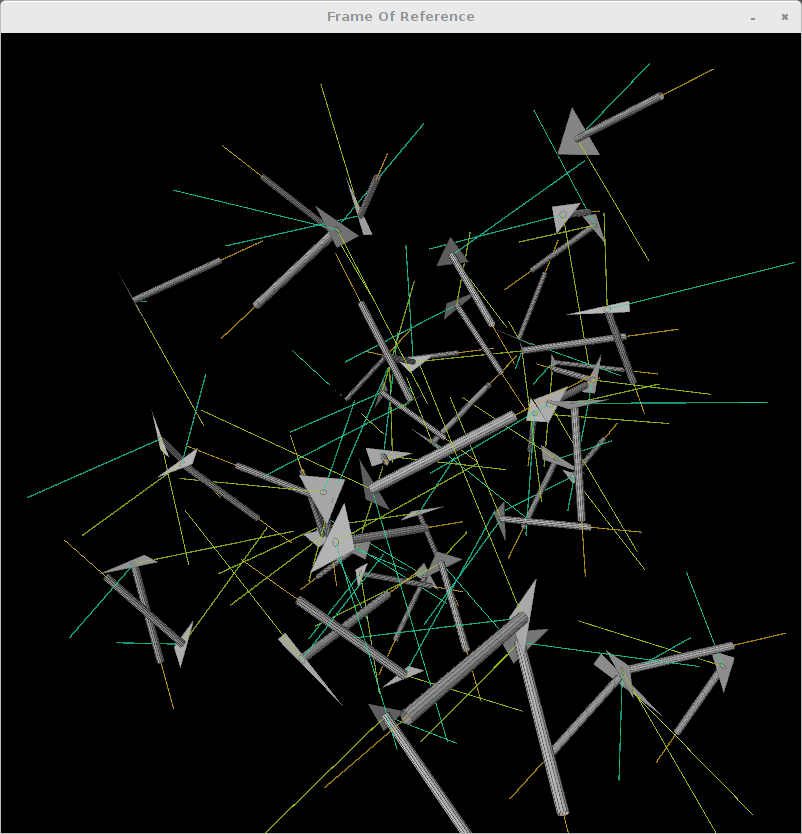Here is vanilla processing sketch by Ira Greenberg translated for JRubyArt. This sketch features the use of ruby Matrix to do 3D transforms it is quite complicated, but is a bit easier on the eye in ruby cf vanilla processing. Also featured are Vec3D (replaces PVector), ArcBall (for intuitive manipulation) and AppRender for efficient Vec3D to vertex translation.

### frame_of_reference.rb

``````###############
# Frame of Reference example by Ira Greenberg
# https://github.com/irajgreenberg/ProcessingTips
# Translated to JRubyArt by Martin Prout December 2015
###############

FACE_COUNT = 50

def settings
size(800, 800, P3D)
end

def setup
sketch_title 'Frame Of Reference'
ArcBall.init(self) # so we use mouse to rotate sketch and mouse wheel to zoom
@renderer = AppRender.new(self) # so we can render Vec3D as vertices
@c = []
@p = []
FACE_COUNT.times do |i|
# calc some random triangles in 3 space
val = Vec3D.new(
rand(-width / 2..width / 2),
rand(-width / 2..width / 2),
rand(-width / 2..width / 2)
)
v0 = Vec3D.new(
rand(-val.x..-val.x + 100),
rand(-val.y..-val.y + 100),
rand(-val.z..-val.z + 100)
)
v1 = Vec3D.new(
rand(-val.x..-val.x + 100),
rand(-val.y..-val.y + 100),
rand(-val.z..-val.z + 100)
)
v2 = Vec3D.new(
rand(-val.x..-val.x + 100),
rand(-val.y..-val.y + 100),
rand(-val.z..-val.z + 100)
)
p << Plane.new([v0, v1, v2])
# build some cute little cylinders
c << Cylinder.new(Vec3D.new(150, 5, 5), 12)
# Using each Triangle normal (N),
# One of the Triangle's edges as a tangent (T)
# Calculate a bi-normal (B) using the cross-product between each N and T
# Note caps represent constants in ruby so we used N = nn, T = tt and B = bb
# in the ruby code below

#
# A picture helps
# nice, sweet orthogonal axes

# N   B
# |  /
# | /
# |/____T

#
# N, T, B together give you a Frame of Reference (cute little local coordinate
# system), based on each triangle. You can then take the cylinder (or any
# vertices) and transform them using a 3 x 3 matrix to this coordinate system.
# (In the matrix each column is based on N, T and B respecivley.)
# The transform will handle any rotations and scaling, but not the translation,
# but we can add another dimenson to the matrix to hold the translation values.
# Here's what all this confusing description looks like:

#
# Matrix :                               Vector :
# |  N.x  T.x  B.x  translation.x  |      |  x  |
# |  N.y  T.y  B.y  translation.y  |      |  y  |
# |  N.z  T.z  B.z  translation.z  |      |  z  |
# |  0    0    0    1              |      |  1  |

# We add the extra row in the matrix and the 1 to each vector
# so the math works. We describe the Matrix as 4 rows by 4 columns
# and the vector now as a Matrix with 4 rows and 1 column.
# When you multiply matrices the inner numbers MUST match, so:
# [4 x 4] [4 x 1] is OK, but [4 x 4] [1 x 4] is NOT COOL.
# see mat4.rb where we use ruby Matrix to handle the multiplication for us

nn = p[i].n
tt = Vec3D.new(
p[i].vecs.x - p[i].vecs.x,
p[i].vecs.y - p[i].vecs.y,
p[i].vecs.z - p[i].vecs.z
)
nn.normalize!
tt.normalize!
bb = nn.cross(tt)
# build matrix with frame and translation (to centroid of each triangle)
m4 = Mat4.new(xaxis: nn, yaxis: tt, zaxis: bb, translate: p[i].c)
# transform each cylinder to align with each triangle
c[i].vecs = m4.mult(c[i].vecs)
end
fill(187)
stroke(50, 20)
end

def draw
background(0)
lights
FACE_COUNT.times do |i|
p[i].display(renderer)
c[i].display(renderer)
end
end
``````

### cylinder.rb

``````class Cylinder
include Processing::Proxy
attr_accessor :vecs

def initialize(dim, detail)
@dim = dim
@detail = detail
init
end

def init
#    created around x-axis
#    y = Math.cos
#    z = Math.sin
veca = []
vecb = []
(0...360).step(360 / detail) do |theta|
cost = DegLut.cos(theta)
sint = DegLut.sin(theta)
veca << Vec3D.new(0, cost * dim.y, sint * dim.z)
vecb << Vec3D.new(dim.x, cost * dim.y, sint * dim.z)
end
@vecs = veca.concat(vecb)
end

def display(renderer)
detail.times do |i|
if i < (detail - 1)
vecs[i].to_vertex(renderer)
vecs[i + 1].to_vertex(renderer)
vecs[detail + i + 1].to_vertex(renderer)
vecs[detail + i].to_vertex(renderer)
else
vecs[i].to_vertex(renderer)
vecs.to_vertex(renderer)
vecs[detail].to_vertex(renderer)
vecs[detail + i].to_vertex(renderer)
end
end
end_shape
end
end
``````

### plane.rb

``````NORM_LEN = 225.0

class Plane
include Processing::Proxy

def initialize(vecs)
@vecs = vecs
init
end

def init
v1 = vecs - vecs
v2 = vecs - vecs

@c = Vec3D.new(
(vecs.x + vecs.x + vecs.x) / 3,
(vecs.y + vecs.y + vecs.y) / 3,
(vecs.z + vecs.z + vecs.z) / 3
)

@n = v1.cross(v2)
n.normalize!
end

def display(renderer)
begin_shape(TRIANGLES)
vecs.map { |vec| vec.to_vertex(renderer) }
end_shape

# normal
stroke(200, 160, 30)
begin_shape(LINES)
c.to_vertex(renderer)
(c + n * NORM_LEN).to_vertex(renderer)
end_shape

# binormal
stroke(160, 200, 30)
begin_shape(LINES)
c.to_vertex(renderer)
# tangent
v = vecs.copy
v -= vecs
v.normalize!
(c + v * NORM_LEN).to_vertex(renderer)
end_shape

stroke(30, 200, 160)
begin_shape(LINES)
c.to_vertex(renderer)
b = v.cross(n)
(c + b * NORM_LEN).to_vertex(renderer)
end_shape
stroke(0, 75)
end
end
``````

### mat4.rb

``````# uber simple Homogeneous 4 x 4 matrix
require 'matrix'

class Mat4

def initialize(xaxis:, yaxis:, zaxis:, translate:)
@mat = Matrix[
[xaxis.x, yaxis.x, zaxis.x, translate.x],
[xaxis.y, yaxis.y, zaxis.y, translate.y],
[xaxis.z, yaxis.z, zaxis.z, translate.z],
[0, 0, 0, 1]
]
end

# Ira's processing version changes the input 'array', here we return
# a new array with transformed values (which we then assign to the input)
# see frame_of_reference.rb

def mult(array)
array.map.each do |arr|
matrix_to_vector(mat * Matrix[[arr.x], [arr.y], [arr.z], ])
end
end

private

# It isn't obvious but we only require first 3 elements
def matrix_to_vector(vec)
Vec3D.new(vec.column(0), vec.column(0), vec.column(0))
end
end
``````

### snapshot of the running sketch